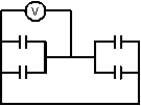Capacitance

# Fundamentals of Physics Study Set 2

Physics & Astronomy

## Quiz 25 :CapacitanceStudy FlashcardsLooking for Introductory Physics Homework Help?## Quiz 25 :Capacitance

Showing 1 - 20 of 59The units of capacitance are equivalent to:
Free
Multiple Choice

EA farad is the same as a:
Free
Multiple Choice

CA parallel-plate capacitor C has a charge Q.The actual charges on its plates are:
Free
Multiple Choice

CEach plate of a capacitor stores a charge of magnitude 1 mC when a 100-V potential difference is applied.The capacitance is:
Multiple ChoiceTo charge a 1-F capacitor with 2 C requires a potential difference of:
Multiple ChoiceIf the charge on a parallel-plate capacitor is doubled:
Multiple ChoiceThe capacitance of a parallel-plate capacitor with plate area A and plate separation d is given by:
Multiple ChoiceThe capacitance of a parallel-plate capacitor is:
Multiple ChoiceThe plate areas and plate separations of five parallel plate capacitors areRank these according to their capacitances, least to greatest.
Multiple ChoiceThe capacitance of a parallel-plate capacitor can be increased by:
Multiple ChoiceIf both the plate area and the plate separation of a parallel-plate capacitor are doubled, the capacitance is:
Multiple ChoiceIf the plate area of an isolated charged parallel-plate capacitor is doubled:
Multiple ChoiceIf the plate separation of an isolated charged parallel-plate capacitor is doubled:
Multiple ChoicePulling the plates of an isolated charged capacitor apart:
Multiple ChoiceA parallel-plate capacitor has a plate area of 0.2 m2 and a plate separation of 0.1 mm.To obtain an electric field of 2.0 106 V/m between the plates, the magnitude of the charge on each plate should be:
Multiple ChoiceA parallel-plate capacitor has a plate area of 0.2 m2 and a plate separation of 0.1 mm.If the charge on each plate has a magnitude of 4 10-6 C the potential difference across the plates is approximately:
Multiple ChoiceThe capacitance of a single isolated spherical conductor with radius R is proportional to:
Multiple ChoiceThe capacitance of a cylindrical capacitor can be increased by:
Multiple ChoiceEach of the four capacitors shown is 500 F.The voltmeter reads 1000V.The magnitude of the charge on each capacitor plate is: